# Grothendieck Group

To give a functor from a category of commutative monoid to the category of abelian group, namely,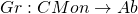let us construct the abelian group for given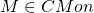, define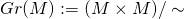with equivalent relation of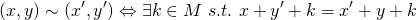and universal property characterized by the following commutative diagram (for given and constructed monoid homomorphism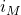and for any monoid homomorphism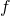, there uniquely exists abelian homomorphism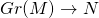that commutes the diagram).By this construction, there’s the identity element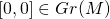and for each element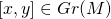we have unique inverse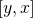and exposes other functorial properties.

To confirm all those properties are valid on this construction, we begin with checking the compatibility of additive operation in the Cartesian product of commutative monoid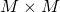and in that of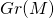, namely,Here q is the quotient map. To verify this, we just show the equation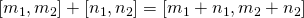for any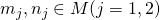is valid.

Let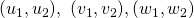represent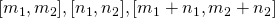respectively. Then the equation above reduces to the equation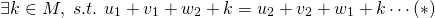Let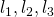be corresponding elements of M to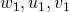in such a way that: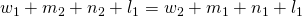,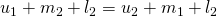and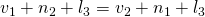. Set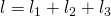and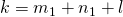, then we have the equation (*) ■

With this compatibility, we can immediately verify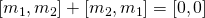.

Now define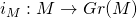by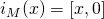. We will now verify the following proposition.

Prop.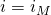is injective if and only if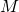has cancellative property, i.e.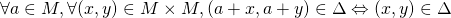Proof.
Suppose M is cancellative, then assuming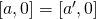is equivalent condition to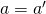. Conversely whenis injective, then if there exists a non-cancellative element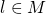for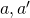, i.e.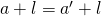but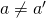.being injective can be restated by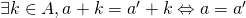, obviously contradict ■

Next let’s show another important property that the Grothendieck construction is idempotent on an abelian group, to be precise, restate in a form of proposition as following.

Prop.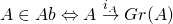is bijective
Proof.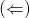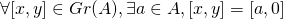. Then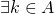s.t.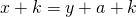. k can be canceled and we have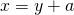. Put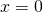, then a is the inverse of y.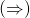Let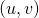represents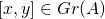. We have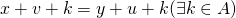. By canceling k, we have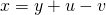, which indicates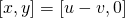#### Another construction:

We cannot avoid another construction of the Grothendieck group by means of completion of group, because not only of the historical importance, but we can also need this for ring construction for the basis of K-theory.

For given, denote the free abelian group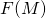generated by the elements of M as the free basis, in which the element is denoted by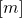for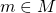. Let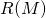be the subgroup ofgenerated by an element of the form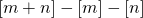. We define the quotient group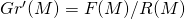.
Our claim is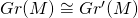.

This isomorphism (and the universal property from the former construction) is verified by proving following proposition.

Prop. The properties of free generator constructed Grothendieck group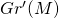.
(a)is composed of a form of element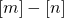.
(b) For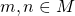,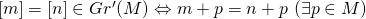Proof.
(a) By expressing the element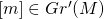as the finite sum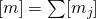, we can immediately get the equation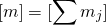And then adding up the differences of generators compose the given form.

(b) When there exists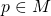s.t.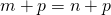, this is obvious by the cancellation of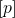. Conversely if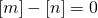, then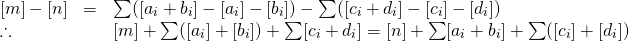Observing the number of generators of free abelian group of the form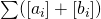and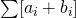, we conclude that we can remove brackets and obtain the results ■

Now consider the following commutative diagram:Here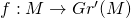is defined by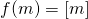, and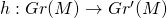is defined by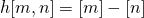.
With the previous proposition, we can see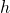is surjective by (a) and injective by (b), then we have.

Finally, we’ll view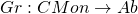as a functor. Let A,B be elements of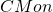. For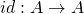,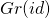is induced identity in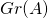because of the uniqueness property:Also the composite property is verified with the uniqueness property by replacing the map and range and extending the diagram above.